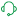# QlikView App Development

Discussion Board for collaboration related to QlikView App Development.

Announcements
Modernize Your QlikView Deployment webinar, Nov. 3rd. REGISTER
cancel
Showing results for
Did you mean:
HighlightedContributor

I have run this script without any errors before. There is literally no change but it is giving me error - field not found 'TOTAL VALUE EUR', 'TOTAL VALUE USD', ... and so on.

Transformed_Detail:
NoConcatenate
[Line #],
[Unit Qty],
[Net Weight (KG)] as [Net Weight (Kg)],
[Part Number] as [Equipment / Part Number],
[Description of Merchandise],
if(isnull([INVOICE CURRENCY]),
[Import
HTS]) as [Import HTS],
if(isnull([INVOICE CURRENCY]),[Export
HTS]) as [Export HTS],

[INVOICE CURRENCY],

[Import
HTS] as [Import HTS for Weight Report],

left(replace(replace([Import
HTS],'-',''),'.',''),8)
as [CN8 Code for Weight Report],
ECCN,
trim(subfield([Country of Origin],'-',1)) as [Country of Origin],

FileName,

if(isnull([INVOICE CURRENCY]),
if(not isnull([TOTAL VALUE EUR]),[TOTAL VALUE EUR]*\$(vEURtoUSD),
if(not isnull([TOTAL VALUE GBP]),[TOTAL VALUE GBP]*\$(vGBPtoUSD),
if(not isnull([TOTAL VALUE USD]),[TOTAL VALUE USD],
if(not isnull([TOTAL VALUE HKD]),[TOTAL VALUE HKD]*\$(vHKDtoUSD),
if(not isnull([TOTAL VALUE AUD]),[TOTAL VALUE AUD]*\$(vAUDtoUSD),
if(not isnull([TOTAL VALUE SGD]),[TOTAL VALUE SGD]*\$(vSGDtoUSD),
if(not isnull([TOTAL VALUE MXN]),[TOTAL VALUE MXN]*\$(vMXNtoUSD),
if(not isnull([TOTAL VALUE NOK]),[TOTAL VALUE NOK]*\$(vNOKtoUSD),
if(not isnull([TOTAL VALUE KRW]),[TOTAL VALUE KRW]*\$(vKRWtoUSD),
if(not isnull([TOTAL VALUE JPY]),[TOTAL VALUE JPY]*\$(vJPYtoUSD),
)))))))))))
,
if([INVOICE CURRENCY]='USD',
[Total Value],[INVOICE CURRENCY])as [Invoice Value USD],

if(isnull([INVOICE CURRENCY]),
if(not isnull([TOTAL VALUE EUR]),[TOTAL VALUE EUR],
if(not isnull([TOTAL VALUE GBP]), ([TOTAL VALUE GBP]*\$(vGBPtoUSD)/\$(vEURtoUSD)),
if(not isnull([TOTAL VALUE USD]),([TOTAL VALUE USD]/\$(vEURtoUSD)))
)),
if([INVOICE CURRENCY]='USD',(
[Total Value]/\$(vEURtoUSD)),[INVOICE CURRENCY])) as [Invoice Value EUR],

if(isnull([INVOICE CURRENCY]),
if(not isnull([TOTAL VALUE EUR]),(([TOTAL VALUE EUR]*\$(vEURtoUSD))/\$(vGBPtoUSD)),
if(not isnull([TOTAL VALUE GBP]), [TOTAL VALUE GBP],
if(not isnull([TOTAL VALUE USD]),([TOTAL VALUE USD]/\$(vGBPtoUSD)))
)),
if([INVOICE CURRENCY]='USD',(
[Total Value]/\$(vGBPtoUSD)),[INVOICE CURRENCY]))
as [Invoice Value GBP],

if(isnull([INVOICE CURRENCY]),
if(not isnull([TOTAL VALUE EUR]),[TOTAL VALUE EUR],
if(not isnull([TOTAL VALUE GBP]), ([TOTAL VALUE GBP]/\$(vEURtoGBP_German)*\$(vEURtoUSD_German)),
if(not isnull([TOTAL VALUE USD]),([TOTAL VALUE USD]/\$(vEURtoUSD_German)))
)),
if([INVOICE CURRENCY]='USD',(
[Total Value]/\$(vEURtoUSD_German)),[INVOICE CURRENCY]))
as [Invoice Value EUR_German],

[Suppl. Unit] as [Supplemental Unit],
[PO / RMA #],
'Item Value' as [Value Type]
RESIDENT Detail;

Labels (4)

• ### Script

3 Replies
HighlightedContributor II

If you comment out this part of the load script and load just 'Detail' do you see those fields still?

If not I would recommend rewriting in the connection line for 'Detail' as I have had excel sheets act up on me sometimes when I connect to them.

HighlightedContributor

Yes I did that and the all the fields load without any error. The script fails when it tries to execute Transformed_Detail part.

HighlightedDigital Support

Kathan, what would be most helpful to me is the actual script log with the failure as well as one where things are successful, so I can see what changes on the failure...

Regards,
Brett

To help users find verified answers, please do not forget to use the "Accept as Solution" button on any post(s) that helped you resolve your problem or question.
I now work a compressed schedule, Tuesday, Wednesday and Thursday, so those will be the days I will reply to any follow-up posts.